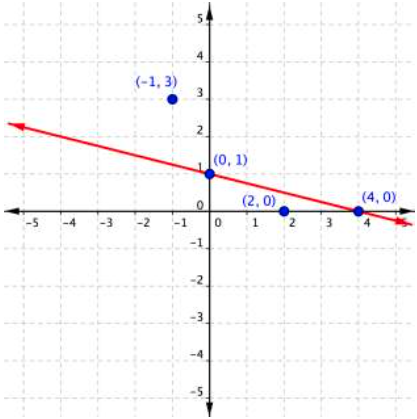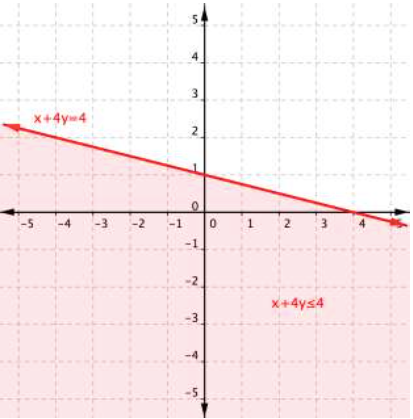# How to graph inequalities

## Graph an Inequality in Two Variables

So how do you get from the algebraic form of an inequality, like $y>3x+1$, to a graph of that inequality? Plotting inequalities is fairly straightforward if you follow a couple steps.

To graph an inequality:

• Graph the related boundary line. Replace the , ≤ or ≥ sign in the inequality with = to find the equation of the boundary line.
• Identify at least one ordered pair on either side of the boundary line and substitute those $(x,y)$ values into the inequality. Shade the region that contains the ordered pairs that make the inequality a true statement.
• If points on the boundary line are solutions, then use a solid line for drawing the boundary line. This will happen for ≤ or ≥ inequalities.
• If points on the boundary line aren’t solutions, then use a dotted line for the boundary line. This will happen for < or > inequalities.

Let’s graph the inequality $x+4y\leq4$.

To graph the boundary line, find at least two values that lie on the line $x+4y=4$. You can use the x– and y-intercepts for this equation by substituting 0 in for x first and finding the value of y; then substitute 0 in for y and find x.

Plot the points $(0,1)$ and $(4,0)$, and draw a line through these two points for the boundary line. The line is solid because ≤ means “less than or equal to,” so all ordered pairs along the line are included in the solution set.The next step is to find the region that contains the solutions. Is it above or below the boundary line? To identify the region where the inequality holds true, you can test a couple of ordered pairs, one on each side of the boundary line.

Related text  How to get bigger legs

If you substitute $(−1,3)$ into $x+4y\leq4$:

$\begin{array}{r}−1+4\left(3\right)\leq4\\−1+12\leq4\\11\leq4\end{array}$

This is a false statement, since 11 is not less than or equal to 4.

On the other hand, if you substitute $(2,0)$ into $x+4y\leq4$:

$\begin{array}{r}2+4\left(0\right)\leq4\\2+0\leq4\\2\leq4\end{array}$

This is true! The region that includes $(2,0)$ should be shaded, as this is the region of solutions.And there you have it—the graph of the set of solutions for $x+4y\leq4$.